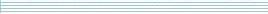| home      | people     | research     | publications    | seminars    | events     | contactCommunication Networks   | Systems Biology   | Hybrid Systems    | Machine Learning   | Dynamics & Interaction### Schools Mathematics Grand Challenge

Week five's Puzzles

Problem 9:

The number 6 has the following property:

If you subtract two from it you get 4 which is a perfect square,

6 - 2 = 4 = (2 x 2) = 2^2

and if you add two to it you get 8 which is a perfect cube,

6 + 2 = 8 = (2 x 2 x 2) = 2^3.

What is the next whole number, strictly bigger than 6, with this property? (meaning that when you subtract two from it, you get a perfect square and when you add two to it, you get a perfect cube)

Problem 10:

The factorial of a positive whole number n is written n! and is calculated by multiplying together all of the whole numbers from n back to one. For instance:

4! = 4x3x2x1 = 24
5! = 5x4x3x2x1 = 120
3! = 3x2x1 = 6
7! = 7x6x5x4x3x2x1 = 5040.

If you add up the factorials of all the whole numbers from 1 up to 1,000,000 (1 million) and divide the sum by 40, what will the remainder be?

So in this question we are asking for the remainder when you divide 1! + 2! + 3! + 4! + 5! + ... + 1,000,000! (the sum of the factorials of the first million positive whole numbers) by 40?

Remember that your answer must be a whole number between 0 and 39.

HINT: You do not need to work out the whole sum. Also be aware that calculators do not always give the right answer when you work with very large numbers!